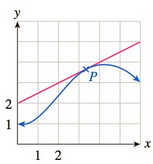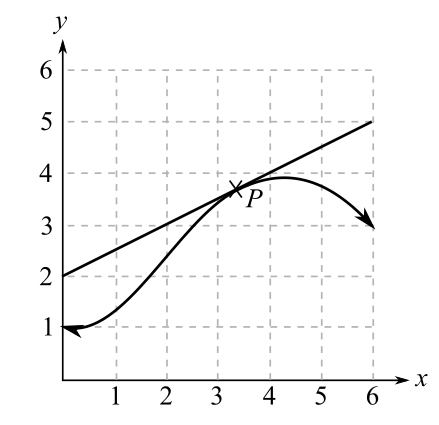Chapter 10.5, Problem 13EFinite Mathematics and Applied Cal...

7th Edition
Stefan Waner + 1 other
ISBN: 9781337274203

Solutions

Chapter
SectionFinite Mathematics and Applied Cal...

7th Edition
Stefan Waner + 1 other
ISBN: 9781337274203
Textbook Problem

In Exercises 13–16 the graph of a function is shown together with the tangent line at a point P. Estimate the derivative of f at the corresponding x value. [HINT: See Quick Example 3.]To determine

To calculate: The derivative of the function f(x) at the corresponding x value from the following graph,Explanation

Given information:

The provided graph is,

Formula used:

The derivative of a function is slope at that point that is m=y2y1x2x1.

Where m is the slope or derivative at that point, (x1,y1) and (x2,y2) are the coordinates.

Calculation:

From the provided graph one can observe that the tangent at point P passes through the coordinates (0,2) and (6,5).

The derivative of the function at point P will be slope of the line at the point

Still sussing out bartleby?

Check out a sample textbook solution.

See a sample solution

The Solution to Your Study Problems

Bartleby provides explanations to thousands of textbook problems written by our experts, many with advanced degrees!

Get Started

In Exercises 19-24, find the functions f + g, f g, fg, and fg. 20. f(x) = x1; g(x) = x3 + 1

Applied Calculus for the Managerial, Life, and Social Sciences: A Brief Approach

True or False: f(x)f(a)xa may be interpreted as the instantaneous velocity of a particle at time a.

Study Guide for Stewart's Single Variable Calculus: Early Transcendentals, 8th

Find for y defined implicity by .

Study Guide for Stewart's Multivariable Calculus, 8th

Find each value of x. log5x=-2

College Algebra (MindTap Course List)